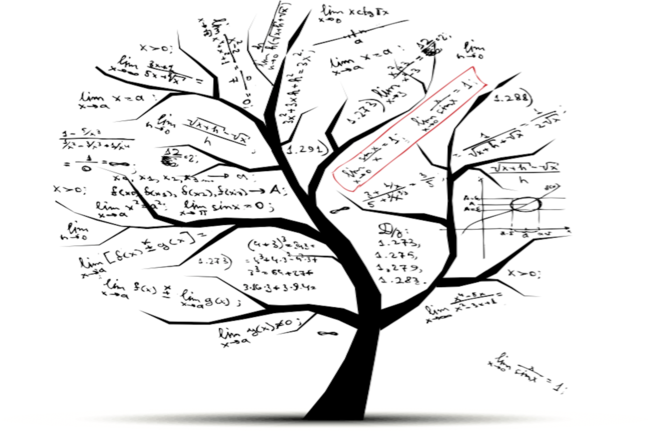3.11

## Weizmann Institute of ScienceThe operator tree

# Operator notation

It is worthwhile mentioning that there are many different notations for operators. The usual notation that we use is to write arithmetic expressions with the operator between the operands: 2 + 3, 3 × 4, 1 + 2 + 3 × 5 etc. This method is called infix.

There are other ways to write expressions. One of them is to write the operator as the first element in the expression, followed by the operands (the numbers). For example, instead of 3 + 4 we write + 3 4. This method is used to write the elementary arithmetic operators (+, -, ×, ÷) and the exponent (denoted ^) and is called prefix, or Polish notation. Since binary operators need exactly two operands, there is no need to write parentheses. It is obvious which operands belong to each operator: each operator operates on the two operands to its right.

It is possible to write complex expressions using this notation: instead of 4 × 2 + 3 we write + 3 × 4 2. Explanation: the addition is performed on the number 3 together with the expression 4 × 2. The result is of course 11. In general, for each operator we check the two operands on its right. If there are expressions (like × 4 2 in the example above) it is performed before performing the operator on the left.

The prefix notation seems complicated at first, but it has two important advantages:

a. It is short and there is no need for parentheses.
b. There is no need to know the order of performing the operations. The order depends on how the expression is written. This makes writing computer software much easier.

There are other ways that we can symbolise operators. Wait a minute! I used the word symbolise. Yes! The operators themselves have a symbology. We use the plus sign and the infix method to symbolise addition but other cultures use different symbols. In Judaism, the plus sign is sometimes written as an inverted T and the division sign is written as a colon (:) i.e. without the ‘bar’. DIV in Excel is QUOTIENT in Numbers. The bra-ket notation is used in quantum mechanics instead of matrix products and integrals.

## What is all this telling us?

That mathematics is a written language. To understand what’s written you need to decipher the symbols that indicate the different elements. We have taken a quick look at the different ways numbers and operators can be written. Of course there are many other mathematical objects, each with their own symbologies. Sets, groups, geometric shapes all have their symbols too. We are getting closer to the end of the course, but before we do, let’s have some ‘operator’ fun…